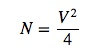In this section, we explain the normalized propagation constant and the waveguide parameter, which are the parameters featuring the optical fiber.

### Normalized propagation constant

Provided the refractive index of core (n1) and clad (n2), and the light propagation angle (n2), mode propagation constant, β, is expressed by the following equation,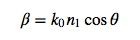where k0=2π/λ is the propagation constant of plane wave in air. By considering the range of propagation anlge, θ<π/2, θ<θc, the possible range of β is given as follows.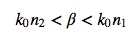Eq.2.2.2 indicates that the propagation constant of waveguide mode can be a value between light wavenumbers in the core and the clad. Although the electric field of waveguide mode is confined and propagated in the core, a part of light is leaked toward the clad. This is possibly why the propagation constant take a value between the propagation constant for the core and the clad. As a normalized parameter of the propagation constant, the normalized propagation constant, b, is defined as follows.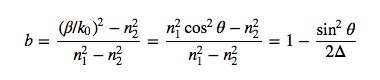### Waveguide parameter

The number of mode possibly existing in optical fiber is determined by the waveguide parameter (V parameter or normalized frequency). The V parameter is defined by the following equation,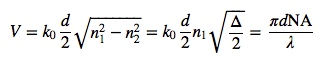where d is the core diameter, NA=sinθmax is the core numerical aperture. The approximation curve for the singlemode dispersion is represented by using the normalized propagation constant, b, and the V parameter, as follows: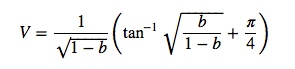The normalized propagation constant and the V parameter for step-type optical fiber is shown as Fig.2.9. LPlm is the linearly polarized mode, and the detail is shown in Fig.2.10. Fig.2.9 is a pattern of LP mode obtained when the linearly polarized light is incident to optical fiber.

At V=2.405, the cut-off occurs, for the propagation constant of LP11 mode equals to 0 (b=0). For V<2.405, only the LP01 mode is propagated (singlemode propagation). For V>2.405, a number of modes are propagated (multimode propagation). This and Eq.8 indicate that the V parameter increases for the relatively shorter wavelength, larger diameter, and larger refractive index difference, and that the number of propagation mode increases.

Fig.2.9 is also used for deriving the propagation constant, β.
By reading the norlamized propagation constant, b, at the V parameter given by Eq., and using Eq.7, the propagation constant, β, is calculated. The propagation constant, β, is used for calculations of the group velocity and its dispersion. For instance, let us consider an SMF with Δ=0.3%, n2=1.460, and d=6.5μm, then, the refractive index, V parameter, normalized propagation constant, and propagation constant of the core at 1030 nm is calculated as n1=1.464,V=2.249,b=0.51,β=8.9×10^6.

The LP mode is an approximated mode, and consists of vector modes (HE, EH, TE, TM). Table2.1 summarizes the relationship between LP modes and the vector modes [144, 143]. Mode degeneration in the waveguide means the state where two or more modes have the same propagation constants with the diiferent electric field distributions. LP01, LP11, and LP21, shown in Fig.2.9, have the similar field distributions as (HE11), (TM01,TE01,HE21), and (HE11,HE31) have, respectively. Fig.2.10 represents the mode pattern for LPlm.

Table 2.1: Relationship between LP modes and the vector modes
 LP modes vector modes degeneracy LP0m HE1m 2 LP1m TE0m, TM0m, HE2m 4 LPlm HEl+1m,EHl−1m 4

For step-type optical fiber, the relationship between the mode number and the V parameter is approximated as follows.